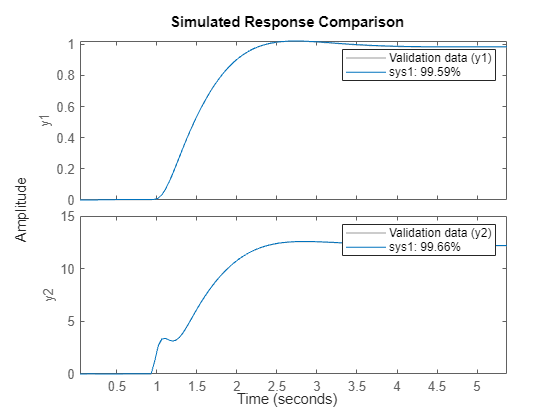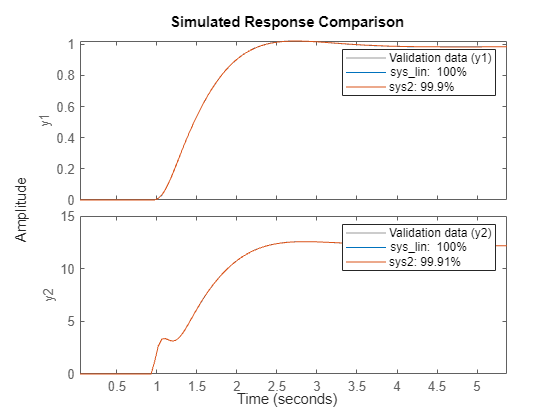## Use State-Space Estimation to Reduce Model Order

Reduce the order of a Simulink® model by linearizing the model and estimating a lower order model that retains model dynamics.

Consider the Simulink model `idF14Model`. Linearizing this model gives a ninth-order model. However, the dynamics of the model can be captured, without compromising the fit quality too much, using a lower-order model.

Obtain the linearized model.

```load_system('idF14Model'); io = getlinio('idF14Model'); sys_lin = linearize('idF14Model',io);```

`sys_lin` is a ninth-order state-space model with two outputs and one input.

Simulate the step response of the linearized model, and use the data to create an `iddata` object.

```Ts = 0.0444; t = (0:Ts:4.44)'; y = step(sys_lin,t); data = iddata([zeros(20,2);y],[zeros(20,1); ones(101,1)],Ts);```

`data` is an `iddata` object that encapsulates the step response of `sys_lin`.

Compare the data to the model linearization.

`compare(data,sys_lin);`Because the data was obtained by simulating the linearized model, there is a complete match between the data and model linearization response.

Identify a state-space model with a reduced order that adequately fits the data.

Determine an optimal model order.

```nx = 1:9; sys1 = ssest(data,nx,'DisturbanceModel','none'); ```

A plot showing the Hankel singular values (SVD) for models of the orders specified by `nx` appears.States with relatively small Hankel singular values can be safely discarded. The plot suggests using a fifth-order model.

At the MATLAB® command prompt, select the model order for the estimated state-space model. Specify the model order as `5`, or press Enter to use the default order value.

Compare the data to the estimated model.

`compare(data,sys1);`The plot displays the fit percentages for the two `sys1` outputs. The four-state reduction in model order results in a relatively small reduction in fit percentage.

Examine the stopping condition for the search algorithm.

`sys1.Report.Termination.WhyStop`
```ans = 'Maximum number of iterations reached.' ```

Create an estimation options set. Specify the `'lm'` search method. Increase the maximum number of search iterations to 50 from the default maximum of 20.

```opt = ssestOptions('SearchMethod','lm'); opt.SearchOptions.MaxIterations = 50; opt.Display = 'on';```

Identify a state-space model using the estimation option set and `sys1` as the estimation initialization model.

`sys2 = ssest(data,sys1,opt);`

Compare the response of the linearized and the estimated models.

`compare(data,sys_lin,sys2);`The updated option set results in better fit percentages for `sys2`.